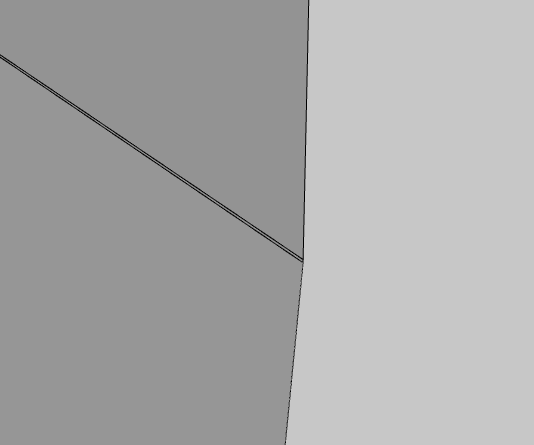# Meshing Tip - Split Periodic Faces

I stumbled across this tip the other day and thought I would share it, as it may be helpful to others.

I use Solidworks for modelling and regularly use the geometry “Check” feature to check for potential problem geometry (especially short edges).

Here is an example of a model that passes the geometry check. There are no edges in this model shorter than 20 mm and it longest edge is 5000 mm. So it’s shortest edge is only 1/250th of its longest edge. I would expect this model to mesh with no problems.

Here is a closer view of the slot in the middle of the plate. Note that the top and bottom ends of the slot are slightly different sizes.

The “fully automatic tetrahedraliztion”" fails on this seemingly simple geometry. Why?

The error reported in the SimScale event log is…

“Mesh could not be computed. A problem occurred in the surface mesh generation.”

But there is a clue listed earlier. SimScale reports the smallest edge being 0.005 mm ,which is much smaller than the expected 20 mm.

It turns out that the short edge is introduced in the STEP file conversion.

In Solidworks there is a STEP export option to “split periodic faces”. When enabled, all cylindrical faces that wrap more than a certain amount (180 degrees I think) are split into smaller faces.

In the example, because the top and bottom rounds of the slot are slightly different sizes, the bottom face wraps slightly more than 180 degrees. In the export, the bottom cylindrical face of the slot is split creating a very small sliver face where the bottom radius meets the vertical sides.

To make matters more interesting Solidworks does not show the sliver face on the exported geometry and the geometry check does not pick it up either. It is, however, visible in the SimScale model viewer.Disabling the “split periodic faces” option solves this problem and the geometry meshes as expected.

Split faces are used to improve the accuracy of geometry, so there may be times when it is advantageous to enable the “split periodic faces option”. For example, a shaft in a bearing. In this case the problem face in this example would need to be split manually at a more logical location.

6 Likes

@BenLewis This is an awesome finding, thank you very much for sharing! I’m sure that other users have already been affected by this problem and your post will definitely save them a lot of time!

Geometry Assembly 1 imported from OnShape also has a small face:

``````Geometry import started.
``````

Number of solids: 17
Number of shells: 17
Number of faces: 874
Number of free faces: 0
Number of wires: 974
Number of edges: 2514
Number of free edges: 0
Number of vertices: 1716
Geometry bounding box diagonal length [m]: 0.0615973
Bounding box x length [m]: 0.0307446
Bounding box y length [m]: 0.0312246
Bounding box z length [m]: 0.04329
Bounding box min-x [m]: -0.0153724
Bounding box min-y [m]: -0.0158623
Bounding box min-z [m]: -0.04
Bounding box max-x [m]: 0.0153721
Bounding box max-y [m]: 0.0153623
Bounding box max-z [m]: 0.00329
Number of small faces: 1 <<<<<<<<<<<<<<<<<<<<<<<<<<<<<<<<<<<<<<<<<<<<<<<<<<<<<
Area of largest face [m²]: 0.000708753
Area of smallest face [m²]: 4.37152e-11
Number of open shells: 0
Geometry is watertight.

And if fails to mesh due to:`Shortest geometry edge length [m]: 2.0397525362e-07 A problem occured in surface mesh generation. Retrying meshing with quadrangle surface elements disabled for the following solid: solid_8 The meshing algorithm failed on the following entities: solid_8_face_5 Mesh could not be computed. A problem occurred in the surface mesh generation.`

There are no options available for whatever conversion is done getting it from there to here, so is there any way to fixing it in simscale?

This video explains how I prepare my geometry for simulation, including identifying and fixing problem geometry. If you’re not using SolidWorks you will need to find the equivalent geometry analysis tools in your CAD package.

2 Likes